# A Counterexample to "An Extension of Gregus Fixed Point Theorem"

## Abstract

In the paper by Olaleru and Akewe (2007), the authors tried to generalize Gregus fixed point theorem. In this paper we give a counterexample on their main statement.

## 1. Introduction

Letbe a Banach space andbe a closed convex subset of. In 1980 Greguš  proved the following results.

Theorem 1.1.

Letbe a mapping satisfying the inequality(1.1)

for all, where, and. Thenhas a unique fixed point.

Several papers have been written on the Gregus fixed point theorem. For example, see . We can combine the Gregus condition by the following inequality, whereis a mapping on metric space: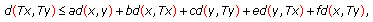(1.2)

for all, where, and.

Definition 1.2.

Letbe a topological vector space on. The mappingis said to be ansuch that for all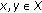(i),

(ii),

(iii),

(iv)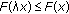for allwith,

(v)ifand, then.

In 2007, Olaleru and Akewe  considered the existence of fixed point ofwhenis defined on a closed convex subsetof a complete metrizable topological vector space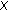and satisfies condition (1.2) and extended the Gregus fixed point.

Theorem 1.3.

Letbe a closed convex subset of a complete metrizable topological vector spaceand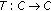a mapping that satisfies(1.3)

for all, whereis anon,, and. Thenhas a unique fixed point.

Here, we give an example to show that the above mentioned theorem is not correct.

## 2. Counterexample

Example 2.1.

Letendowed with the Euclidean metric and. Letdefined by. Letandsuch that. Then for allsuch that, we have that(2.1)

We have two cases,or.

If, then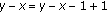, and hence inequality (2.1) is true. If, then, and so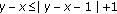, and hence inequality (2.1) is true. So condition (1.3) holds for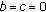and, buthas not fixed point.

## References

1. 1.

Greguš M Jr.: A fixed point theorem in Banach space. Unione Matematica Italiana. Bollettino. A 1980,17(1):193–198.

2. 2.

Ćirić LjB: On a generalization of a Greguš fixed point theorem. Czechoslovak Mathematical Journal 2000,50(3):449–458. 10.1023/A:1022870007274

3. 3.

Fisher B, Sessa S: On a fixed point theorem of Greguš. International Journal of Mathematics and Mathematical Sciences 1986,9(1):23–28. 10.1155/S0161171286000030

4. 4.

Jungck G: On a fixed point theorem of Fisher and Sessa. International Journal of Mathematics and Mathematical Sciences 1990,13(3):497–500. 10.1155/S0161171290000710

5. 5.

Mukherjee RN, Verma V: A note on a fixed point theorem of Greguš. Mathematica Japonica 1988,33(5):745–749.

6. 6.

Murthy PP, Cho YJ, Fisher B: Common fixed points of Greguš type mappings. Glasnik Matematički. Serija III 1995,30(2):335–341.

7. 7.

Olaleru JO, Akewe H: An extension of Gregus fixed point theorem. Fixed Point Theory and Applications 2007, 2007:-8.

Authors

## Rights and permissions

Reprints and Permissions

Moradi, S. A Counterexample to "An Extension of Gregus Fixed Point Theorem". Fixed Point Theory Appl 2011, 484717 (2011). https://doi.org/10.1155/2011/484717